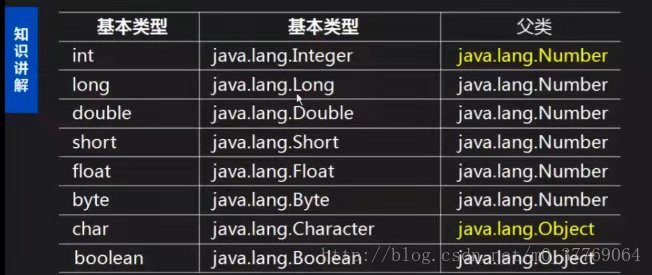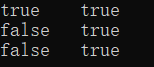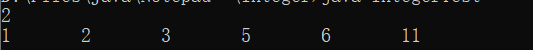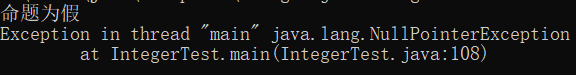• ## 包装类

千次阅读 多人点赞 2019-02-24 21:42:49
包装类(Wrapper Class): Java语言是一个面向对象的语言，但是Java中的基本数据类型却是不面向对象的。基本类型的数据不具备&amp;amp;amp;quot;对象&amp;amp;amp;quot;的特性（没有成员变量和成员方法可以...
一.包装类： 1. 包装类(Wrapper Class):

Java语言是一个面向对象的语言，但是Java中的基本数据类型却是不面向对象的。基本类型的数据不具备"对象"的特性（没有成员变量和成员方法可以调用），因此，java为每种数据类型分别设计了对应的类，即包装类。

基本数据类型对应的包装类byteByteshortShortintIntegerlongLongcharCharacterfloarFloatdoubleDoublebooleanBoolean
2.包装类的特点：（1）所有包装类都是final类型，因此不能创建他们的子类。 （2）包装类是不可变类，一个包装类的对象自创建后，他所包含的基本类型数据就不能被改变。

二.Integer类
1.装箱和拆箱

装箱：将基本数据类型变为包装类对象。 拆箱：将包装类中包装的基本数据类型取出。

int到IntegerInteger到int使用Integer类的构造方法调用Integer类的intValue()方法使用Integer类内部的valueOf( )方法自动拆箱自动装箱
2.int到nteger类：

三种方法： （１）使用Integer类的构造方法 （２）使用Integer类内部的valueOf( )方法 （３）自动装箱

（1）方法1：使用Integer类的构造方法

public Integer(int value)：将int类型转换为Integer类。public Integer(String s)：将String类型转换为Integer类。 注意：使用此构造方法时，字符串内的字符必须由数字组成，不可以是字母或者其他形式，否则抛出NumberFormatException。
public static void main(String[] args)
{
//方式1:public Integer(int value)
int i= 100;
//将int类型的i转换为Integer类型的ii
Integer ii = new Integer(i);
System.out.println("ii:"+ii);

//方式２:public Integer(String s)
String s = "100";
//String s = "abc";出错,因为这个字符串必须由数字字符组成。
Integer iii = new Integer(s);
System.out.println("iii:"+iii);//100
}

（２）方法2:使用Integer类内部的valueOf方法
public static Integer valueOf(int i)：将int类型转换为Integer类。
Integer i3 = Integer.valueOf(30);

（３）方法3:自动装箱的方法: 事实上，编译器自动执行了valueOf方法。
 Integer ii = 100;//编译器自动执行了Integer ii = Integer.valueOf(100)

包装类装箱拆箱BytevalueOf()byteValue()ShortvalueOf()shortValue()IntegervalueOf()intValue()LongvalueOf()longValue()FloatvalueOf()floatValue()DoublevalueOf()doubleValue()CharactervalueOf()charValue()BooleanvalueOf()booleanValue()
valueOf源码分析：
public static Integer valueOf(int i) {
assert IntegerCache.high>= 127;
if (i >= IntegerCache.low&& i <= IntegerCache.high)
return IntegerCache.cache[i+ (-IntegerCache.low)];
return new Integer(i);
}

通过查看原码可知，java针对-128-127之间的数据做了一个数据缓冲池。 如果数据是该范围内的，每次并不创建新的空间。 如果数据是该范围内的，就new一个空间
相关面试题：
public static void main(String[] args)
{
Integer i1 = new Integer(127);
Integer i2 = new Integer(127);
System.out.println(i1 == i2);//false
System.out.println(i1.equals(i2));//true重写了equals方法，比较的内容

Integer i3 = new Integer(128);
Integer i4 = new Integer(128);
System.out.println(i3 == i4);//false
System.out.println(i3.equals(i4));//true

Integer i5 = 128;
Integer i6 = 128;
System.out.println(i5 == i6);//false
System.out.println(i5.equals(i6));//true

Integer i7 = 127;
Integer i8 = 127;
System.out.println(i7 == i8);//true
System.out.println(i7.equals(i8));//true


3.Integer类到 int:

（１）调用包装类的intValue()方法 （２）通过自动拆箱：

方法1:调用包装类的intValue()方法 public int intValue()：以 int 类型返回该 Integer 的值。
public void test2() {
Integer i1 = new Integer(14);
Float f1 = new Float(12.3F);
//1.调用包装类的XxxValue()方法
int i2 = i1.intValue();//i2 = 14
float f2 = f1.floatValue();//f2 = 12.3
}

方法2:通过自动拆箱： 编译器自动执行了valueOf方法
        //5是基本数据类型，通过自动装箱变成对象类型。
//编译器执行了Integer iii = Integer.valueOf(5)
Integer iii=5;
//自动拆箱，实际上执行了 int iii2 = iii.intValue()
int iii2=iii;
System.out.println(iii2);

4.int类型数据和String形式之间的相互转换：
intl到StringString到int字符串的拼接String–>Integer–>intString类的valueOf()方法int parseInt(String s)int --> Integer -->String (所用方法：valueOf()+toString())toString(int i)
public static void main(String[] args)
{
//int--String把int类型的数据转化为字符串的形式
int number = 100;

//方式１:字符串的拼接
String s1 = "" + number;
System.out.println("s1:"+s1);

//方式２:String类的valueOf可以把任意类型的数据转换成String类型
String s2 = String.valueOf(number);
System.out.println("s2:"+s2);

//方式3
//int -- Integer -- String
Integer i = new Integer(number);
String s3 = i.toString();
System.out.println("s3:"+s3);

//方式4
//public static String toString(int i)返回一个表示该 Integer 值的 String 对象。
String s4 = Integer.toString(number);
System.out.println("s4:"+s4);

//String--int
String s ="100";
//方式１
//String--Integer--int
//String--Integer
Integer ii = new Integer(s);
//Integer--int
//public int intValue()以 int类型返回该 Integer的值
int x = ii.intValue();
System.out.println("x:"+x);

//方式２
//public static int parseInt(String s)
int y = ii.parseInt(s);
System.out.println("y:"+y);
}

5.进制的转换：
public static void main(String[] args)
{
//十进制到二进制，八进制，十六进制
System.out.println(Integer.toBinaryString(100));
System.out.println(Integer.toOctalString(100));
System.out.println(Integer.toHexString(100));
System.out.println("--------");

//十进制到其他进制
System.out.println(Integer.toString(100,10));
System.out.println(Integer.toString(100,2));
System.out.println(Integer.toString(100,8));
System.out.println(Integer.toString(100,15));
System.out.println(Integer.toString(100,5));
System.out.println(Integer.toString(100,7));
System.out.println(Integer.toString(100,-7));
System.out.println(Integer.toString(100,70));
System.out.println(Integer.toString(100,34));
System.out.println(Integer.toString(100,37));
System.out.println(Integer.toString(100,36));

//其他进制转换为十进制
System.out.println(Integer.parseInt("100",10));
System.out.println(Integer.parseInt("100",2));
System.out.println(Integer.parseInt("100",4));
System.out.println(Integer.parseInt("100",16));
System.out.println(Integer.parseInt("100",23));
//System.out.println(Integer.parseInt("123",2));出错，因为2进制没有3
}

三.Character类 1.Character类概述：
Character类在对象中包装了一个char的值,用于对单个字符进行操作。 该类提供了几种方法来操纵字符，以确定字符的类别（小写字母，数字，等等），并将字符从大写转换成小写等。
2.Character类的构造方法：
public Character(char value)：将char类型转换为Character类。
Character ch1 = new Character('a');
System.out.println("ch1:"+ch1);  //ch1:97

注意：char和Character类之间的转换方法与int和Integer类之间的转换方法相同，不再赘述。 3.Character类的方法：
序号方法说明1isDigit()是否是一个数字字符2isWhiteSpace()是否是一个空格3isUpperCase()是否是大写字母4isLowerCase()是否是小写字母5toUpperCase()指定字母的大写形式6toLowerCase()指定字母的小写形式7toString()返回字符的字符串形式
public static void main(String[] args)
{
//public static boolean isUpperCase(int codePoint)判断给定的字符是否为大写字符
System.out.println(Character.isUpperCase('A'));
System.out.println(Character.isUpperCase('a'));
System.out.println(Character.isUpperCase('0'));

//public static boolean isLowerCase(char ch):判断给定的字符是否是小写字符
System.out.println(Character.isUpperCase('A'));
System.out.println(Character.isUpperCase('a'));
System.out.println(Character.isUpperCase('0'));

// public static boolean isDigit(int codePoint):判断给定的字符是否是数字
System.out.println(Character.isUpperCase('A'));
System.out.println(Character.isUpperCase('a'));
System.out.println(Character.isUpperCase('0'));

//public static char toUpperCase(char ch):把给定的字符转换为大写字符
System.out.println(Character.toUpperCase('a'));

//public static char toLowerCase(char ch):把给定的字符转换为小写字符
System.out.println(Character.toLowerCase('A'));
}


展开全文• 包装类均位于java.lang包，八种包装类和基本数据类型的对应关系如表 在这八个类名中， 除了Integer和Character类以外，其它六个类的类名和基本数据类型一致，只是类名的第一个字母大写而已 。 在这八个类中，除了...
Java是面向对象的语言，但并不是“纯面向对象”的，因为我们经常用到的基本数据类型就不是对象。但是我们在实际应用中经常需要将基本数据转化成对象，以便于操作。比如：将基本数据类型存储到Object[]数组或集合中的操作等等。为了解决这个不足，Java在设计类时为每个基本数据类型设计了一个对应的类进行代表，这样八个和基本数据类型对应的类统称为包装类(Wrapper Class)。包装类均位于java.lang包，八种包装类和基本数据类型的对应关系如表在这八个类名中，除了Integer和Character类以外，其它六个类的类名和基本数据类型一致，只是类名的第一个字母大写而已。在这八个类中，除了Character和Boolean以外，其他的都是“数字型”，“数字型”都是java.lang.Number的子类**。Number类是抽象类，因此它的抽象方法**，所有子类都需要提供实现。Number类提供了抽象方法：intValue()、longValue()、floatValue()、doubleValue()，意味着所有的**“数字型”包装类都可以互相转型**。下面我们通过一个简单的示例认识一下包装类。
public class WrapperClassTest {
public static void main(String[] args) {
Integer i = new Integer(10);
Integer j = new Integer(50);
}
}

内存分析如图展开全文java
• ## Java中的包装类

万次阅读 多人点赞 2019-04-16 15:34:10
目录 ...七、包装类使用过程中可能引起的空指针异常 八、为什么需要包装类包装类又为什么要保留基本数据类型？（包装类的优缺点） 一、包装类概述 Java8种基本数据类型：整型(byt...
目录
一、包装类概述
二、包装类的自动装箱、自动拆箱机制
三、包装类中的缓存机制
四、包装类的四则运算、位运算、比较运算、逻辑运算
五、包装类作为方法的形参、返回值
六、包装类作为集合的元素
七、包装类使用过程中有可能引起的空指针异常
八、为什么需要包装类？有了包装类又为什么要保留基本数据类型？（包装类的优缺点）

一、包装类概述
Java有8种基本数据类型：整型(byte、short、int、long)、浮点型(float、double)、布尔型boolean、字符型char，相对应地，Java提供了8种包装类Byte、Short、Integer、Long、Float、Double、Boolean、Character。包装类创建对象的方式就跟其他类一样。
Integer num = new Integer(0);    //创建一个数值为0的Integer对象
二、包装类的自动装箱、自动拆箱机制
上面的构造对象语句实际上是基本数据类型向包装类的转换。在应用中我们经常需要进行基本类型数据和包装类对象之间的互转。
Integer num1 = new Integer(1);	//基本数据类型转为包装类
int num2 = num1.intValue();		//包装类型转为基本数据类型
System.out.println(num1 +"	"+ num2);
而Java为了方便我们使用，以及出于其他目的如性能调优，给我们提供了自动装箱、拆箱机制。这种机制简化了基本类型和包装类型的转换。
//1、包装类中的自动装箱拆箱机制
Integer  num1 = 1;		//自动装箱
int num2 = num1;		//自动拆箱
System.out.println(num1 +"	"+ num2);
Integer integer = Integer.valueOf(1);
int i = integer.intValue();
System.out.println((new StringBuilder()).append(integer).append("\t").append(i).toString());
可见，Java编译器帮我们完成了转换操作。另外，我们可以看到，除了使用new关键字，还可以使用Integer类的valueOf()方法创建一个Integer对象。这两个方式是有所区别的，我们下面会说到。
三、包装类中的缓存机制
前面说到创建包装类对象有两种方式：new关键字、valueOf()方法。我们来看一段代码感受一下它们的区别。
//2、包装类中的缓存机制
Integer num3 = 10;
Integer num4 = 10;
Integer num5 = new Integer(20);
Integer num6 = new Integer(20);
Integer num7 = 128;
Integer num8 = 128;
System.out.println((num3==num4) +"	"+ num3.equals(num4));
System.out.println((num5==num6) +"	"+ num5.equals(num6));
System.out.println((num7==num8) +"	"+ num7.equals(num8));
运行结果为我们看下它的反编译代码
Integer integer = Integer.valueOf(10);
Integer integer1 = Integer.valueOf(10);
Integer integer2 = new Integer(20);
Integer integer3 = new Integer(20);
Integer integer4 = Integer.valueOf(128);
Integer integer5 = Integer.valueOf(128);
System.out.println((new StringBuilder()).append(integer == integer1).append("\t").append(integer.equals(integer1)).toString());
System.out.println((new StringBuilder()).append(integer2 == integer3).append("\t").append(integer2.equals(integer3)).toString());
System.out.println((new StringBuilder()).append(integer4 == integer5).append("\t").append(integer4.equals(integer5)).toString());
首先，我们查看Integer的valueOf()方法的源码
    public static Integer valueOf(int i) {
if (i >= IntegerCache.low && i <= IntegerCache.high)
return IntegerCache.cache[i + (-IntegerCache.low)];
return new Integer(i);
}
再查看下Integer的内部类IntegerCache的cache数组成员、low、high成员
        static final int low = -128;
static final int high;
static final Integer cache[];

static {
// high value may be configured by property
int h = 127;
String integerCacheHighPropValue =
sun.misc.VM.getSavedProperty("java.lang.Integer.IntegerCache.high");
if (integerCacheHighPropValue != null) {
try {
int i = parseInt(integerCacheHighPropValue);
i = Math.max(i, 127);
// Maximum array size is Integer.MAX_VALUE
h = Math.min(i, Integer.MAX_VALUE - (-low) -1);
} catch( NumberFormatException nfe) {
// If the property cannot be parsed into an int, ignore it.
}
}
high = h;

cache = new Integer[(high - low) + 1];
int j = low;
for(int k = 0; k < cache.length; k++)
cache[k] = new Integer(j++);

// range [-128, 127] must be interned (JLS7 5.1.7)
assert IntegerCache.high >= 127;
}
可以发现，只要Integer类第一次被使用到，Integer的静态内部类就被加载，加载的时候会创建-128到127的Integer对象，同时创建一个数组cache来缓存这些对象。当使用valueOf()方法创建对象时，就直接返回已经缓存的对象，也就是说不会再新建对象；当使用new关键字or使用valueOf()方法创建小于-128大于127的值对象时，就会创建新对象。
//2、包装类中的缓存机制
Integer num3 = 10;
Integer num4 = 10;
Integer num5 = new Integer(20);
Integer num6 = new Integer(20);
Integer num7 = 128;
Integer num8 = 128;

由于num3、num4都小于等于127，它们指向的是同一个缓存的Integer对象，所以用==进行比较的结果是true；num5、num6由于使用new关键字指向的是两个不同的新对象，结果为false；num7、num8虽然是采用自动装箱的方式，但执行valueOf()方法的时候，由于不满足条件i >= IntegerCache.low && i <= IntegerCache.high，而同样新建了两个不同的新对象，结果同样是false。
接着，我们再来看看源码中Integer的equals()方法的实现
    public boolean equals(Object obj) {
if (obj instanceof Integer) {
return value == ((Integer)obj).intValue();
}
return false;
}
可见equals()方法比较的是Integer对象的值，而不是像==一样比较的是对象是否是同一个对象。所以，当需要比较两个Integer对象的值是否相等时，记住要用equals()方法。用==比较的话由于缓存机制的存在，可能产生一些让人困扰的结果。
此外，在8种包装类型中，有缓存区的有Character、Byte、Short、Integer、Long，而且它们的实现方式基本一样，都是-128到127的缓存范围。Boolean虽然没有缓存区，但是因为只有两个值true、false，所以Boolean在成员变量中就创建了两个相应的对象。没有缓存区的只有Float、Double，之所以没有原因很简单，即便是0到1这么小的范围，浮点数也有无数个，使用缓存区缓存它们不具备可能性和实用性。
缓存区的存在使得常用的包装类对象可以得到复用，这有利于提升性能。当我们需要创建新对象的时候再new一个，增加了灵活性。
四、包装类的四则运算、位运算、比较运算、逻辑运算
1、四则运算和位运算
//四则运算、位运算
Integer num9 = 1;
Integer num10 = 2;
Integer num11 = num9 + num10;
Short num12 = 5;
Integer num13 = num9 + num12;
Long num14 = num9 + 10L;
System.out.println(num9 << 1);	//位运算
System.out.println(num9 +"	"+ num10 +"	"+ num11 +"	"+ num12 +"	"+ num13 +"	"+ num14);反编译结果如下
        Integer integer = Integer.valueOf(1);
Integer integer1 = Integer.valueOf(2);
Integer integer2 = Integer.valueOf(integer.intValue() + integer1.intValue());
Short short1 = Short.valueOf((short)5);
Integer integer3 = Integer.valueOf(integer.intValue() + short1.shortValue());
Long long1 = Long.valueOf((long)integer.intValue() + 10L);
System.out.println(integer.intValue() << 1);
System.out.println((new StringBuilder()).append(integer).append("\t").append(integer1).append("\t").append(integer2).append("\t").append(short1).append("\t").append(integer3).append("\t").append(long1).toString());

可以看到Integer num11 = num9 + num10; 这一句被划分为3个步骤：将两个Integer对象分别进行拆箱；将拆箱得到的两个int数值相加求其和；将和值进行装箱，从而将num11指向缓存数组中值为3的Integer对象。
而Short num12 = 5; 这一句则先将5强制转换成short类型，再将其装箱把值为5的Short对象的引用赋给num12。
而Integer num13 = num9 + num12; 这一句除了Integer num11 = num9 + num10;的3个步骤，中间还有short+int=int的类型自动提升的过程。
而Long num14 = num9 + 10L; 这一句Integer num11 = num9 + num10;的3个步骤，中间还有强制类型转换的过程。需要注意的是，如果是Long num14 = num9 + num10; 的话就会出现类型不匹配的错误，因为num9、num10拆箱之后相加的和是int类型，而Long.valueOf(long)需要的形参是long类型，自然会出错。我们也可以看到，当包装类型对象和基本类型数据进行四则运算的时候，对象是会被拆箱的，然后再按基本类型数据的运算规则进行运算。
另外，如果仅仅是打印两个包装类型对象求和的结果，是不会有将和值重新转换成该包装类型的步骤的，如下面所示
System.out.println(num9 + num10);
System.out.println(integer.intValue() + integer1.intValue());
这里还需要注意一点，尽管基本类型与自动类型提升/强制类型转换，包装类是没有类似的用法的。下面的做法是错的。
Short num3 = 10;
Integer num4 = num3;	//错误: 不兼容的类型: Short无法转换为Integer
Long num5 = (Long)num4;	//错误: 不兼容的类型: Integer无法转换为Long
Double num6 = num5;	//错误: 不兼容的类型: Long无法转换为Double
小结：不同包装类型对象是不能直接转换的，不过有两种途径可以代替：一种是上面讨论的不同包装类对象进行四则运算后赋给某一种类型；另一种就是利用包装类的方法
Integer a = 20;
Long b = a.longValue();
Short c = b.shortValue();
System.out.println(a +"	"+ b +"	"+ c);
2、比较运算和逻辑运算
Integer num9 = 100;
Integer num10 = 200;
Short num11 = 50;
Long num12 = 50L;
System.out.println((num9<num10) +"	"+ (num9<200) +"	"+ (num9<num11) +"	"+ (num9<num12) +"	"+ (num9<10L));
反编译结果为
Integer integer = Integer.valueOf(100);
Integer integer1 = Integer.valueOf(200);
Short short1 = Short.valueOf((short)50);
Long long1 = Long.valueOf(50L);
System.out.println((new StringBuilder()).append(integer.intValue() < integer1.intValue()).append("\t").append(integer.intValue() < 200).append("\t").append(integer.intValue() < short1.shortValue()).append("\t").append((long)integer.intValue() < long1.longValue()).append("\t").append((long)integer.intValue() < 10L).toString());

可以看到，两个同类型的包装类对象进行比较时比较的其实是各自的基本类型数值，如num9 < num10；两个不同类型的包装类对象进行比较时则在比较基本类型数值之前，会有类型提升or强制类型转换，如num9 < num11，num9 < num12。
当想比较两个对象是否相等时，注意要使用equals()方法，从前面的讨论也知道，使用==的话比较的其实是引用的对象是否同一个，一般不满足我们的需求。
Integer num13 = new Integer(100);
System.out.println(num9.equals(num13) +"	"+ num9.equals(50));
反编译结果为
Integer integer2 = new Integer(100);
System.out.println((new StringBuilder()).append(integer.equals(integer2)).append("\t").append(integer.equals(Integer.valueOf(50))).toString());

逻辑运算举例：
System.out.println((num9&1));
反编译结果为
System.out.println(integer.intValue() & 1);
五、包装类作为方法的形参、返回值
//包装类作为方法的形参、返回值
public static Integer intToInteger(int i) {
return i;
}
public static int integerToInt(Integer i) {
return i;
}
反编译结果为
    public static Integer intToInteger(int i)
{
return Integer.valueOf(i);
}

public static int integerToInt(Integer integer)
{
return integer.intValue();
}
六、包装类作为集合的元素
//包装类作为集合元素
List list = new ArrayList();
Iterator it = list.iterator();
while (it.hasNext()) {
System.out.println(it.next());
}
反编译结果为

ArrayList arraylist = new ArrayList();
for(Iterator iterator = arraylist.iterator(); iterator.hasNext(); System.out.println(iterator.next()));
                List<Integer> list = new ArrayList<>();
for (int i=0; i<5; i++) {
}
Iterator it = list.iterator();
while (it.hasNext()) {
System.out.println(it.next());
}
反编译结果为
        ArrayList arraylist = new ArrayList();
for(int i = 0; i < 5; i++)

for(Iterator iterator = arraylist.iterator(); iterator.hasNext(); System.out.println(iterator.next()));
七、包装类使用过程中有可能引起的空指针异常
//注意包装类可能产生的空引用异常
Boolean flag1 = false;
System.out.println(flag1?"命题为真":"命题为假");
Boolean flag2 = null;
System.out.println(flag2?"命题为真":"命题为假");
Boolean flag3 = true;
运行结果为这里只是简单演示空指针异常。平时使用时需要注意这一点，比如当Boolean的对象作为形参时，在方法执行体的头部需要做下null检测。
上述代码的反编译结果为
        Boolean boolean1 = Boolean.valueOf(false);
System.out.println(boolean1.booleanValue() ? "\u547D\u9898\u4E3A\u771F" : "\u547D\u9898\u4E3A\u5047");
Boolean boolean2 = null;
System.out.println(boolean2.booleanValue() ? "\u547D\u9898\u4E3A\u771F" : "\u547D\u9898\u4E3A\u5047");
Boolean boolean3 = Boolean.valueOf(true);
可见三目运算符的条件表达式的位置一定是boolean值，如果你传入的是Boolean对象，则会自动拆箱转换为boolean值。
另外，三目运算符的其他两个表达式位置也是如此，会把包装类对象转换为相应的基本类型对象。
八、为什么需要包装类？有了包装类又为什么要保留基本数据类型？（包装类的优缺点）
为什么需要包装类？
首先，Java语言是一个面向对象的语言，但是Java中的基本数据类型却是不面向对象的，将每个基本数据类型设计一个对应的类进行代表，这种方式增强了Java面向对象的性质。
其次，如果仅仅有基本数据类型，那么在实际使用时将存在很多的不便，很多地方都需要使用对象而不是基本数据类型。比如，在集合类中，我们是无法将int 、double等类型放进去的，因为集合的容器要求元素是Object类型。而包装类型的存在使得向集合中传入数值成为可能，包装类的存在弥补了基本数据类型的不足。
此外，包装类还为基本类型添加了属性和方法，丰富了基本类型的操作。如当我们想知道int取值范围的最小值，我们需要通过运算，如下面所示，但是有了包装类，我们可以直接使用Integer.MAX_VALUE即可。
//求int的最大值
int max = 0;
int flag = 1;
for (int i=0; i<31; i++) {
max += flag;
flag = flag << 1;
}
System.out.println(max +"	"+ Integer.MAX_VALUE); //2147483647      2147483647
为什么要保留基本数据类型？
我们都知道在Java语言中，用new关键字创建的对象是存储在堆里的，我们通过栈中的引用来使用这些对象，所以，对象本身来说是比较消耗资源的。对于经常用到的类型，如int等，如果我们每次使用这种变量的时候都需要new一个对象的话，就会比较笨重了。所以，Java提供了基本数据类型，这种数据的变量不需要使用new在堆上创建，而是直接在栈内存中存储，因此会更加高效。

展开全文自动装箱
• ## 【面向对象】包装类

万次阅读 多人点赞 2017-10-24 11:32:07
包装类概念，包装类的用途，
一、什么是包装类
包装类（Wrapper Class）： Java是一个面向对象的编程语言，但是Java中的八种基本数据类型却是不面向对象的，为了使用方便和解决这个不足，在设计类时为每个基本数据类型设计了一个对应的类进行代表，这样八种基本数据类型对应的类统称为包装类(Wrapper Class)，包装类均位于java.lang包。
二、包装类的用途
为了使用方便Java中将8中基本数据类型进行了封装：除了Integer和Character类以后，其它六个类的类名和基本数据类型一直，只是类名的第一个字母大写即可。
boolean —> Booleanchar —> Characterbyte—> Byteshort—> Shortlong—> Longint —> Integerfloat—> Floatdouble—> Double
对于包装类说，用途主要包含两种： a、作为 和基本数据类型对应的类 类型存在，方便涉及到对象的操作。 b、包含每种基本数据类型的相关属性如最大值、最小值等，以及相关的操作方法。
三、包装类的实际使用（以int和integer为例）
1.int和integer类之间的转换 在实际转换时，使用Integer类的构造方法和Integer类内部的intValue方法实现这些类型之间的相互转换：
		//
int n=5;
Integer n1=new Integer(n);
System.out.println("int类型转换为integer类："+n1);
//
Integer i=new Integer(50);
int i1 = i.intValue();
System.out.println("integer类转换为int类型："+i1);

2、Integer类内部的常用方法 Integer类的主要方法有：parseInt方法和toString方法。
		//parseInt方法： 数字字符串类型转成int类型
String ss="123";
int ii = Integer.parseInt(ss);
System.out.println("字符类型转成整型："+ii);
//toString方法：int类型转成数字字符串类型
int ii2=123;
String ss2 = Integer.toString(ii2);
System.out.println("int类型转成数字字符串类型:"+ss);

JDK自从1.5版本以后，就引入了自动拆装箱的语法，也就是在进行基本数据类型和对应的包装类转换时，系统将自动进行，这将大大方便程序员的代码书写。 自动装箱：将 基本数据类型 封装为对象类型，来符合java的面向对象的思想。 自动拆箱：将对象重新转化为基本数据类型。
		//5是基本数据类型，通过自动装箱变成对象类型。
//编译器执行了Integer iii = Integer.valueOf(5)
Integer iii=5;
//自动拆箱，实际上执行了 int iii2 = iii.intValue()
int iii2=iii;
System.out.println(iii2);

valueOf（）源码分析：
public static Integer valueOf(int i) {
assert IntegerCache.high>= 127;
if (i >= IntegerCache.low&& i <= IntegerCache.high)
return IntegerCache.cache[i+ (-IntegerCache.low)];
return new Integer(i);
}

说明：Integer iii=5;相当于编译器执行了Integer iii = Integer.valueOf(5)操作。这个方法就是返回一个 Integer 对象，只是在返回之前，看作了一个判断，判断当前 i 的值是否在 [-128,127] 区别，且 IntegerCache 中是否存在此对象，如果存在，则直接返回引用，否则，创建一个新的对象。
3.包装类的缓存值 各个包装类缓存值范围 ：
boolean：true和false
byte：-128～127
char：0～127
short：-128～127
int：-128～127
long：-128～127

特别注意：对于float和double没有缓存。 在对上述值进行装箱的时候（如：Character c1=127;or Integer i= Integer.valueOf(1); 这个不行哦：Integer i2=new Integer(1)；因为这是新建了一个对象出来。）,并不是创建一个新对象而是使用缓存中的对象，如果超出范围则需要新建立对象。
四、常见的面试题
1.Java中Int与Integer的区别？
一、int 是基本类型，直接存数值；而integer引用数据类型。
二、Int的声明不需要实例化，且变量声明后的初始值为0；Integer的是一个类，初始值为null，需要进行实例化，才能对变量数据进行处理。
三、Integer类是int的包装类，实际开发中Integer被看成一个对象，可以进行数据转换等操作。

2.判断下面程序片段输出的结果：
        Integer num1 = 200;
Integer num2 = 200;
System.out.println("num1==num2: "+(num1==num2));
Integer num3 = 100;
Integer num4 = 100;
System.out.println("num3==num4: "+(num3==num4));

输出结果：num1num2:false 和num3num4:true 说明：
首先要明确equal方法与的区别： equals() 比较的是两个对象的值（内容）是否相同。 "" 比较的是两个对象的引用（内存地址）是否相同，也用来比较两个基本数据类型的变量值是否相等。java定义：在自动装箱时对于值从–128到127之间的值，它们被装箱为Integer对象后，会存在内存中被重用，始终只存在一个对象而如果超过了从–128到127之间的值，被装箱后的Integer对象并不会被重用，即相当于每次装箱时都新建一个 Integer对象；
展开全文Java面向对象
• 最近在业务中碰到了 Integer 默认值的判空 导致的业务逻辑问题，引起了兴趣，针对基本数据类型和其包装类有什么区别？测试过程中应该注意哪些问题呢？...回答这些问题前，我们先来看看java中基本数据类型和包装类有哪些测试 java 基本数据类型
• ## JAVA常用类之包装类

千次阅读 2015-12-27 12:44:13
每个JAVA基本类型在java.lang包中都一个相应的包装类。 基本类型 包装类 boolean Boolean byte Byte char Character short Short int Integer long Long float Float double Doublejava
• ## Java中包装类作用及注意点

万次阅读 多人点赞 2018-03-16 19:30:50
Java中对每种基本类型都一个对应的包装类，这里主要讲解包装类的作用和包装类使用时的一些注意点。 包装类的作用 作用主要以下两方面： - 编码过程中只接收对象的情况，比如List中只能存入对象，不能存入...equals
• ## 18 - JavaScript 包装类

千次阅读 2019-10-23 23:04:05
包装类 包装类的介绍 我们都知道，js中的数据类型包括以下几种。 基本数据类型：String、Number、Boolean、Null、Undefined 引用数据类型：Object JS为我们提供了三个包装类： String()：将基本数据类型字符...
• Java8种基本数据类型，为什么又要出现对应的8种包装类：1、Java的8种基本数据类型不支持面向对象编程机制2、8种基本数据类型不具备“对象”的特性：没有成员变量、方法可供调用3、例如：某个方法需要Object类型的...
• ## 包装类和基本类型

万次阅读 多人点赞 2019-03-06 09:18:53
包装类有以下用途 1.集合不允许存放基本数据类型，故常用包装类 2.包含了每种基本类型的相关属性，如最大值，最小值，所占位数等 3.作为基本数据类型对应的类类型，提供了一系列实用的对象操作，如类型转换，进制...
• 包装类及常用方法简介 JAVA 是一种面向对象语言，JAVA 中的类把方法与数据连接在一起，构成 了自包含式的处理单元。但在 JAVA 中不能定义基本类型（primitive type)对象， 为了能将基本类型视为对象进行处理，并...Java
• ## 包装类Wrapper Class

千次阅读 2018-08-07 12:07:57
为什么需要包装类？ Java并不是纯面向对象的语言，Java语言是一个面向对象的语言，但是Java中的基本数据类型确实不面向对象的，但是我们在实际使用中经常需要将基本数据转化成对象，便于操作，比如：集合的操作中...
• ## Java包装类的共同点

千次阅读 2019-03-10 23:19:32
渣渣海除了写点博客外，还有github哦...之前写过一篇博客，讲了基础的Java包装类的装包拆包过程，下面补充一下包装类它们的一些共同点。 1.所有包装类都重写了Object方法 我们也很容易知道Object的方法： bo...
• 包装类的共性 数据类型转换 基本数据类型 序号 类型名称 默认值 大小 最小值 最大值 包装类 缓冲区间 1 boolean false 1B 0(false) 1(true) Boolean 无 2 byte...Java基础 基本数据类型
• 包装类  我们对基本数据类型都非常熟悉，例如int、float、double、boolean、char等。基本数据类型是不具备对象的特性 的，比如基本类型不能调用方法、功能简单。为了让基本数据类型也具备对象的特性，Java为每...JavaSE java
• ## Java数据类型—包装类

万次阅读 多人点赞 2020-12-16 16:16:27
1. 为什么需要包装类：JAVA是面向对象的语言，很多类和方法中的参数都需使用对象(例如集合)，但基本数据类型却不是面向对象的，这就造成了很多不便 2. 拆装箱的概念：将基本数据类型转为包装类的过程叫装箱，将包装...java jvm
• ## Java 包装类是什么

万次阅读 多人点赞 2018-04-26 14:29:49
包装类就是将基本的数据类型以及一些辅助方法封装到类中，例如 class IntDemo { private int num; public IntDemo(int num) { this.num = num; } public int intValue() { return this.num; } } Java...拆箱与装箱
• Double 在对象中包装了一个基本类型 double 的值。Double 对象包含一个 double 类型的字段。此外，该还提供了多个方法，可以将 double 类型与 String 类型相互转换，同时 还提供了处理 double 类型时比较常用...Java Java类
• 这个问题的重心不仅仅在于考察对包装类存在合理性的认识，也在考察对基本数据类型存在意义的理解。 我们都知道在Java语言中，new一个对象存储在堆里，我们通过栈中的引用来使用这些对象。但是对于经常用到的一系列类...基本类型 Java
• 区别： 1.基本类型存储在栈里，包装类型存储在堆里。因为栈的效率更高，所以保留了基本类型。 2.包装类是对象，拥有方法和字段，对象的调用是引用对象的地址。... 基本类型，包装类 以及其默认值,包装...基本类型 默认值
• java基本数据类型对象包装类   一、包装类说明 为了方便操作基本数据类型值，将其封装成了对象，在对象中定义了属性和行为丰富了该数据的操作。用于描述该对象的类就称为基本数据类型对象包装类。 基本数据类型与...java
• java的基本数据类型都一个对应的包装类，所对应的类都有哪些对应的常用方法java
• ## JAVA中包装类的作用

万次阅读 多人点赞 2019-04-16 12:04:37
包装类（Wrapper Class）： Java是一个面向对象的编程语言，但是Java中的八种基本数据类型却是不面向对象的，为了使用方便和解决这个不足，在设计类时为每个基本数据类型设计了一个对应的类进行代表，这样八种基本...
• ## JS 包装类

千次阅读 2018-07-28 10:31:23
JS中只有对象才属性和方法，原始值没有属性和方法 那么我们平常求字符串的长度 用length属性不是没有问题吗？ var str=&amp;amp;amp;amp;amp;quot;hello world&amp;amp;amp;amp;amp;quot;; var lon
•java
• ## java中基本类型包装类

万次阅读 多人点赞 2020-12-14 08:38:00
1. 基本类型包装类概述为了方便操作基本数据类型值，将其封装成了对象，在对象中定义了属性和行为丰富了该数据的操作。用于描述该对象的类就称为基本数据类型对象包装类。将基本数据类型封装成对象...字符串 java 编程语言 eclipse css...